407 493 6601

# 7th Grade - Area of Paralleogram

## Introduction

• A parallelogram is a special quadrilateral (four sides) with two pairs of parallel sides.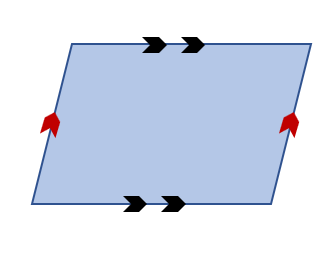• The opposite sides of a parallelogram are equal in length.
• The opposite angles of a parallelogram are equal in measure.
• The sum of all the interior angles of a parallelogram is equal to $360°$.

## Area of Parallelogram

• The area of a parallelogram is the region enclosed by it.
• If we know the length of the base and height of a parallelogram, we can determine its area by using the formula mentioned below.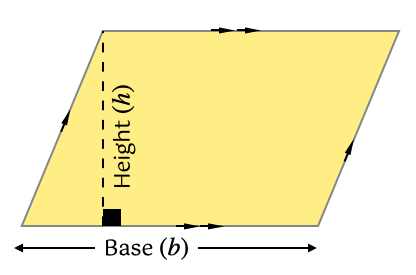, where $b=$length of the base, and $h=$height of the parallelogram

## Solved Examples

Question 1: Find the area of the parallelogram shown below.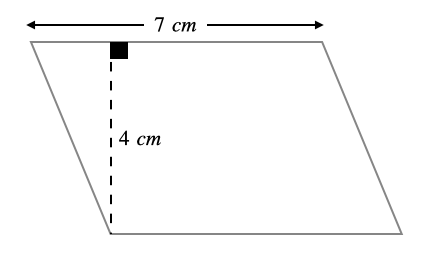Solution:  $=7×4$

Question 2: Find the area of the parallelogram shown below.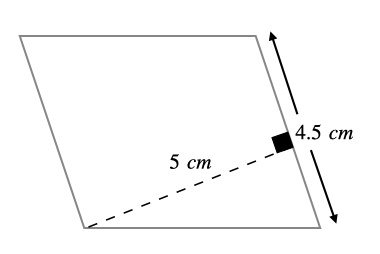Solution:  $=4.5×5$

Question 3: Find the area of the parallelogram shown below.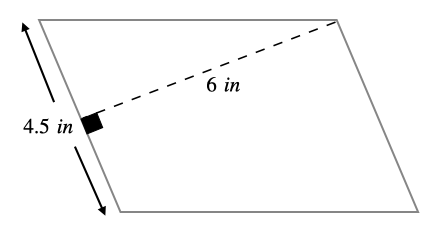Solution:  $=4.5×6$

## Cheat Sheet

• , where $b=$length of the base, and $h=$height of the parallelogram.
• Any side of a parallelogram can be chosen as the base of the parallelogram.
• The perpendicular dropped on the base from the opposite vertex is known as height (altitude).

## Blunder Areas

• All the quadrilaterals are not parallelograms.